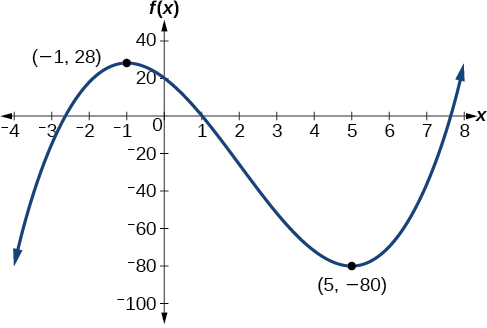3.3 Rates of change and behavior of graphs  (Page 4/15)

 Page 4 / 15

Finding increasing and decreasing intervals on a graph

Given the function $\text{\hspace{0.17em}}p\left(t\right)\text{\hspace{0.17em}}$ in [link] , identify the intervals on which the function appears to be increasing.

We see that the function is not constant on any interval. The function is increasing where it slants upward as we move to the right and decreasing where it slants downward as we move to the right. The function appears to be increasing from $\text{\hspace{0.17em}}t=1\text{\hspace{0.17em}}$ to $\text{\hspace{0.17em}}t=3\text{\hspace{0.17em}}$ and from $\text{\hspace{0.17em}}t=4\text{\hspace{0.17em}}$ on.

In interval notation    , we would say the function appears to be increasing on the interval (1,3) and the interval $\left(4,\infty \right).$

Finding local extrema from a graph

Graph the function $\text{\hspace{0.17em}}f\left(x\right)=\frac{2}{x}+\frac{x}{3}.\text{\hspace{0.17em}}$ Then use the graph to estimate the local extrema of the function and to determine the intervals on which the function is increasing.

Using technology, we find that the graph of the function looks like that in [link] . It appears there is a low point, or local minimum, between $\text{\hspace{0.17em}}x=2\text{\hspace{0.17em}}$ and $\text{\hspace{0.17em}}x=3,\text{\hspace{0.17em}}$ and a mirror-image high point, or local maximum, somewhere between $\text{\hspace{0.17em}}x=-3\text{\hspace{0.17em}}$ and $\text{\hspace{0.17em}}x=-2.$

Graph the function $\text{\hspace{0.17em}}f\left(x\right)={x}^{3}-6{x}^{2}-15x+20\text{\hspace{0.17em}}$ to estimate the local extrema of the function. Use these to determine the intervals on which the function is increasing and decreasing.

The local maximum appears to occur at $\text{\hspace{0.17em}}\left(-1,28\right),\text{\hspace{0.17em}}$ and the local minimum occurs at $\text{\hspace{0.17em}}\left(5,-80\right).\text{\hspace{0.17em}}$ The function is increasing on $\text{\hspace{0.17em}}\left(-\infty ,-1\right)\cup \left(5,\infty \right)\text{\hspace{0.17em}}$ and decreasing on $\text{\hspace{0.17em}}\left(-1,5\right).$Finding local maxima and minima from a graph

For the function $\text{\hspace{0.17em}}f\text{\hspace{0.17em}}$ whose graph is shown in [link] , find all local maxima and minima.

Observe the graph of $\text{\hspace{0.17em}}f.\text{\hspace{0.17em}}$ The graph attains a local maximum at $\text{\hspace{0.17em}}x=1\text{\hspace{0.17em}}$ because it is the highest point in an open interval around $\text{\hspace{0.17em}}x=1.$ The local maximum is the $\text{\hspace{0.17em}}y$ -coordinate at $\text{\hspace{0.17em}}x=1,\text{\hspace{0.17em}}$ which is $\text{\hspace{0.17em}}2.$

The graph attains a local minimum at because it is the lowest point in an open interval around $\text{\hspace{0.17em}}x=-1.\text{\hspace{0.17em}}$ The local minimum is the y -coordinate at $\text{\hspace{0.17em}}\text{\hspace{0.17em}}x=-1,\text{\hspace{0.17em}}\text{\hspace{0.17em}}$ which is $\text{\hspace{0.17em}}\text{\hspace{0.17em}}-2.$

Analyzing the toolkit functions for increasing or decreasing intervals

We will now return to our toolkit functions and discuss their graphical behavior in [link] , [link] , and [link] .

Use a graph to locate the absolute maximum and absolute minimum

There is a difference between locating the highest and lowest points on a graph in a region around an open interval (locally) and locating the highest and lowest points on the graph for the entire domain. The $\text{\hspace{0.17em}}y\text{-}$ coordinates (output) at the highest and lowest points are called the absolute maximum and absolute minimum , respectively.

To locate absolute maxima and minima from a graph, we need to observe the graph to determine where the graph attains it highest and lowest points on the domain of the function. See [link] .

Not every function has an absolute maximum or minimum value. The toolkit function $\text{\hspace{0.17em}}f\left(x\right)={x}^{3}\text{\hspace{0.17em}}$ is one such function.

Absolute maxima and minima

The absolute maximum    of $\text{\hspace{0.17em}}f\text{\hspace{0.17em}}$ at $\text{\hspace{0.17em}}x=c\text{\hspace{0.17em}}$ is $\text{\hspace{0.17em}}f\left(c\right)\text{\hspace{0.17em}}$ where $\text{\hspace{0.17em}}f\left(c\right)\ge f\left(x\right)\text{\hspace{0.17em}}$ for all $\text{\hspace{0.17em}}x\text{\hspace{0.17em}}$ in the domain of $\text{\hspace{0.17em}}f.$

The absolute minimum    of $\text{\hspace{0.17em}}f\text{\hspace{0.17em}}$ at $\text{\hspace{0.17em}}x=d\text{\hspace{0.17em}}$ is $\text{\hspace{0.17em}}f\left(d\right)\text{\hspace{0.17em}}$ where $\text{\hspace{0.17em}}f\left(d\right)\le f\left(x\right)\text{\hspace{0.17em}}$ for all $\text{\hspace{0.17em}}x\text{\hspace{0.17em}}$ in the domain of $\text{\hspace{0.17em}}f.$

Finding absolute maxima and minima from a graph

For the function $\text{\hspace{0.17em}}f\text{\hspace{0.17em}}$ shown in [link] , find all absolute maxima and minima.

Observe the graph of $\text{\hspace{0.17em}}f.\text{\hspace{0.17em}}$ The graph attains an absolute maximum in two locations, $\text{\hspace{0.17em}}x=-2\text{\hspace{0.17em}}$ and $\text{\hspace{0.17em}}x=2,\text{\hspace{0.17em}}$ because at these locations, the graph attains its highest point on the domain of the function. The absolute maximum is the y -coordinate at $\text{\hspace{0.17em}}x=-2\text{\hspace{0.17em}}$ and $\text{\hspace{0.17em}}x=2,\text{\hspace{0.17em}}$ which is $\text{\hspace{0.17em}}16.$

The graph attains an absolute minimum at $\text{\hspace{0.17em}}x=3,\text{\hspace{0.17em}}$ because it is the lowest point on the domain of the function’s graph. The absolute minimum is the y -coordinate at $\text{\hspace{0.17em}}x=3,$ which is $-10.$

sin^4+sin^2=1, prove that tan^2-tan^4+1=0
what is the formula used for this question? "Jamal wants to save \$54,000 for a down payment on a home. How much will he need to invest in an account with 8.2% APR, compounding daily, in order to reach his goal in 5 years?"
i don't need help solving it I just need a memory jogger please.
Kuz
A = P(1 + r/n) ^rt
Dale
how to solve an expression when equal to zero
its a very simple
Kavita
gave your expression then i solve
Kavita
Hy guys, I have a problem when it comes on solving equations and expressions, can you help me 😭😭
Thuli
Tomorrow its an revision on factorising and Simplifying...
Thuli
ok sent the quiz
kurash
send
Kavita
Hi
Masum
What is the value of log-1
Masum
the value of log1=0
Kavita
Log(-1)
Masum
What is the value of i^i
Masum
log -1 is 1.36
kurash
No
Masum
no I m right
Kavita
No sister.
Masum
no I m right
Kavita
tan20°×tan30°×tan45°×tan50°×tan60°×tan70°
jaldi batao
Joju
Find the value of x between 0degree and 360 degree which satisfy the equation 3sinx =tanx
what is sine?
what is the standard form of 1
1×10^0
Akugry
Evalute exponential functions
30
Shani
The sides of a triangle are three consecutive natural number numbers and it's largest angle is twice the smallest one. determine the sides of a triangle
Will be with you shortly
Inkoom
3, 4, 5 principle from geo? sounds like a 90 and 2 45's to me that my answer
Neese
answer is 2, 3, 4
Gaurav
prove that [a+b, b+c, c+a]= 2[a b c]
can't prove
Akugry
i can prove [a+b+b+c+c+a]=2[a+b+c]
this is simple
Akugry
hi
Stormzy
x exposant 4 + 4 x exposant 3 + 8 exposant 2 + 4 x + 1 = 0
x exposent4+4x exposent3+8x exposent2+4x+1=0
HERVE
How can I solve for a domain and a codomains in a given function?
ranges
EDWIN
Thank you I mean range sir.
Oliver
proof for set theory
don't you know?
Inkoom
find to nearest one decimal place of centimeter the length of an arc of circle of radius length 12.5cm and subtending of centeral angle 1.6rad
factoring polynomialByByBy